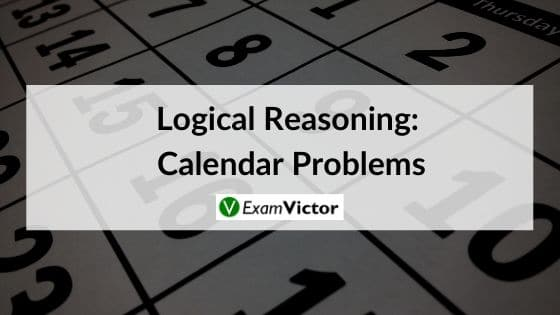# Logical Reasoning: Calendar ProblemsLast Updated on Nov 9, 2020

Calendar problems always seem to be confusing for the students. In this article, we are going to discuss some tips and tricks to easily solve the calendar problems.

Important terms:

Odd Days: In a given period, the number of days more than the complete weeks are called odd days.

Leap Year:
(i)Every year is divisible by 4 is a leap year, if it is not a century
(ii)Every 4th century is a leap year and no other century is a leap year.
Note: A leap year has 366 days and the month February has 29 days

Ordinary Year: The year which is not a leap year is called an ordinary year. An ordinary year has 365 days and the month February has 28 days.

Counting of Odd Days :
(i)1 leap year has 366 days = 52 weeks + 2 days.
So, 1 leap year has 2 odd days

(ii) 1 ordinary year = 365 days = 52 weeks + 1 day.
So, 1 ordinary year has 1 odd day

100 years = 76 ordinary years + 24 leap years
= 76X1 + 24X2 = 76 + 48 = 124 days
= 17 weeks + 5 days = 5 odd days
SO, 100 years has 5 odd days

Number of odd days in 100 years = 5
Number of odd days in 200 years = 3
Number of odd days in 300 years = 1
Number of odd days in 400 years = 0

Similarly, each one of 800 years, 1200 years, 1600 years and 2000 years etc has 0 odd days.

Days of the week related to odd days:
0 odd days —> Sunday
1 odd day —> Monday
2 odd days —> Tuesday
3 odd days —> Wednesday
4 odd days —> Thursday
5 odd days —> Friday
6 odd days —> Saturday

Example.

What was the day on 15th august 1947 ?

(a) Friday
(b)Saturday
(c) Sunday
(d)Thursday

Solution-
15 Aug, 1947 = (1946 years + Period from 1.1.1947 to 15.8.1947)
Odd days in 1600 years = 0
Odd days in 300 years = 1
46 years = (35 ordinary years + 11 leap years) = (35 x 1 + 11 x 2)= 57 (8 weeks + 1 day) = 1 odd day

Jan. Feb. Mar. Apr. May. Jun. Jul. Aug
( 31 + 28 + 31 + 30 + 31 + 30 + 31 + 15 ) = 227 days = (32 weeks + 3 days) = 3 odd days.

Total number of odd days = (0 + 1 + 1 + 3) = 5 odd days.
Hence, as the number of odd days = 5, given day is Friday.

### Summary

In this blog post, we have taken up one of the most confusing topics for students ‘ Calendar Problems’. We have explained this topic right from the beginning so that the base is prepared and then we have taken some examples with detailed solutions. We hope you liked our post. For related posts click here. Do not forget to share your views in the comment section below.